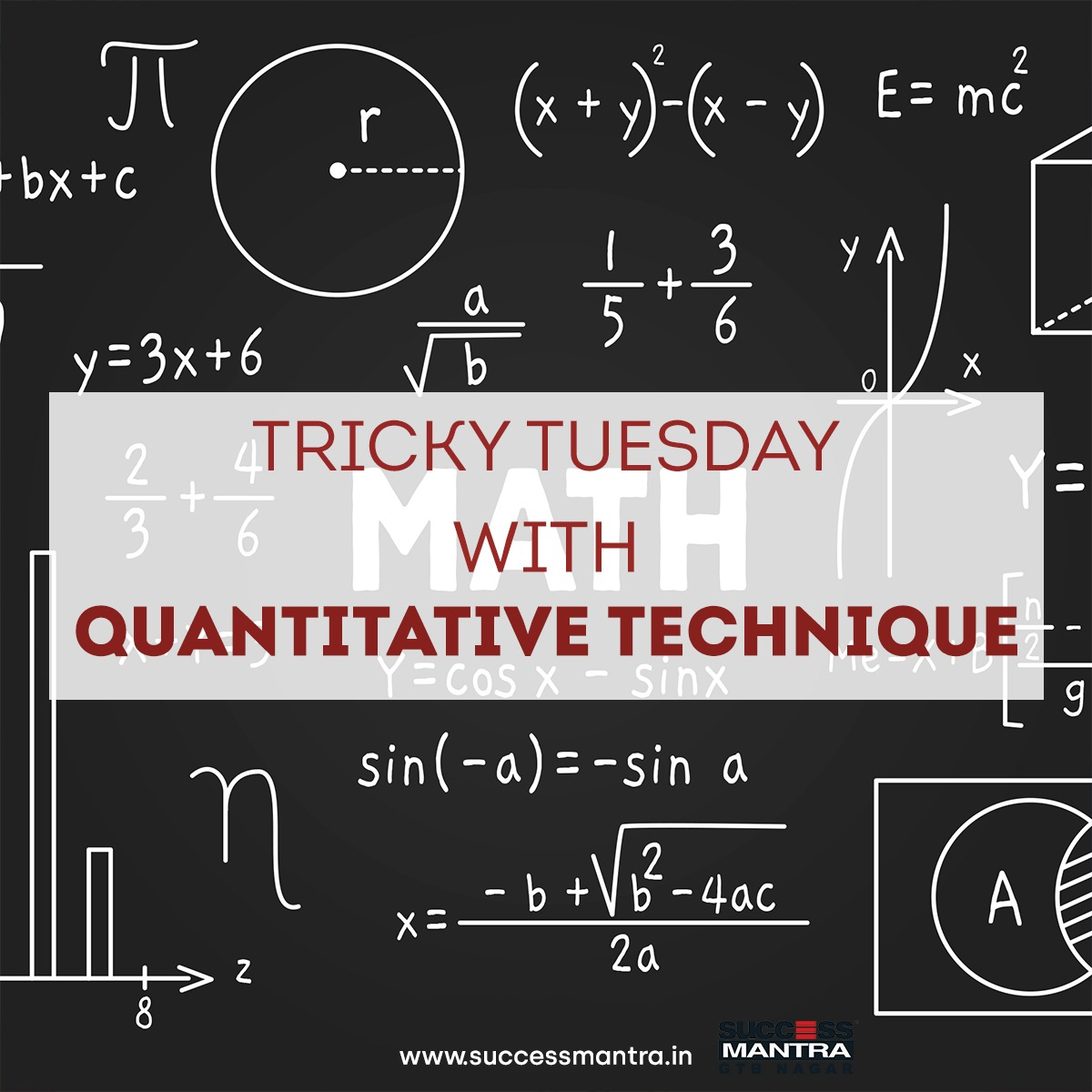## Questions On Quantitative Techniques SMQTQ0211. The product of 2 numbers is 1575 and their quotient is 9/7. Then the sum of the numbers is –

a. 74
b. 78
c. 80
d. 90

Ans : C

2. √6+√6+√6+… is equal to –

a. 2
b. 5
c. 4
d. 3

Ans : D

3. The sum of the squares of two natural consecutive odd numbers is 394. The sum of the numbers is –

a. 24
b. 32
c. 40
d. 28

Ans : D

4. When (6767 +67) is divided by 68, the remainder is-

a. 1
b. 63
c. 66
d. 67

Ans : C

5. If 738 A6A is divisible by 11, then the value of A is-

a. 6
b. 3
c. 9
d. 1

Ans : C